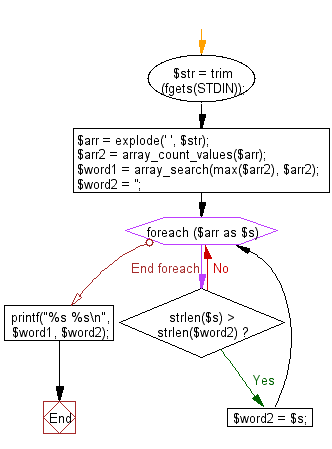﻿ PHP Exercise: Reads a text and prints two words - w3resource

# PHP Exercises: Reads a text and prints two words

## PHP: Exercise-61 with Solution

Write a PHP program which reads a text (only alphabetical characters and spaces.) and prints two words. The first one is the word which is arise most frequently in the text. The second one is the word which has the maximum number of letters.
Note: A word is a sequence of letters which is separated by the spaces.

Input:A text is given in a line with following condition:
a. The number of letters in the text is less than or equal to 1000.
b. The number of letters in a word is less than or equal to 32.
c. There is only one word which is arise most frequently in given text.
d. There is only one word which has the maximum number of letters in given text.

Sample Solution: -

PHP Code:

``````<?php
\$str = trim(fgets(STDIN));
\$arr = explode(' ', \$str);
\$arr2 = array_count_values(\$arr);
\$word1 = array_search(max(\$arr2), \$arr2);
\$word2 = '';
foreach (\$arr as \$s) {
if (strlen(\$s) > strlen(\$word2)) {
\$word2 = \$s;
}
}
printf("%s %s\n", \$word1, \$word2);
?>
```
```

Sample Input:

Sample Output:

`your participation.`

Flowchart:PHP Code Editor:

Have another way to solve this solution? Contribute your code (and comments) through Disqus.

What is the difficulty level of this exercise?

Test your Programming skills with w3resource's quiz.

﻿

## PHP: Tips of the Day

Mutates the original array to filter out the values specified

Example:

```<?php
function tips_pull(&\$items, ...\$params)
{
\$items = array_values(array_diff(\$items, \$params));
return \$items;
}

\$items = ['x', 'y', 'z', 'x', 'y', 'z'];
print_r(tips_pull(\$items, 'y', 'z'));
?>
```

Output:

```Array
(
 => x
 => x
)
```